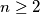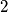### MEMO 2018 ekipno problem 2

Kvaliteta:
Avg: 0.0
Težina:
Avg: 6.0

Let$P$ be a polynomial of degree$n \geq 2$ with rational coefficients such that$P$ has$n$ pairwise distinct real roots forming an arithmetic progression. Prove that among the roots of$P$ there are two that are also roots of some degree$2$ polynomial with rational coefficients.

Source: Srednjoeuropska matematička olimpijada 2018, ekipno natjecanje, problem 2